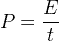Electric power

Electric power is the rate of energy consumption in an electrical circuit.

The electric power is measured in units of watts.

Electric power definition

The electric power P is equal to the energy consumption E divided by the consumption time t:P is the electric power in watt (W).

E is the energy consumption in joule (J).

t is the time in seconds (s).

Example

Find the electric power of an electrical circuit that consumes 120 joules for 20 seconds.

Solution:

E = 120J

t = 20s

P = E / t = 120J / 20s = 6W

Electric power calculation

P = V I

or

P = I 2 R

or

P = V 2 / R

P is the electric power in watt (W).

V is the voltage in volts (V).

I is the current in amps (A).

R is the resistance in ohms (Ω).

Power of AC circuits

The formulas are for single phase AC power.

For 3 phase AC power:

When line to line voltage (VL-L) is used in the formula, multiply the single phase power by square root of 3 (√3=1.73).

When line to zero voltage (VL-0) is used in the formula, multiply the single phase power by 3.

Real power

Real or true power is the power that is used to do the work on the load.

P = Vrms Irms cos φ

P      is the real power in watts [W]

Vrms  is the rms voltage = Vpeak/√2 in Volts [V]

Irms   is the rms current = Ipeak/√2 in Amperes [A]

φ      is the impedance phase angle = phase difference between voltage and current.

Reactive power

Reactive power is the power that is wasted and not used to do work on the load.

Q = Vrms Irms sin φ

Q      is the reactive power in volt-ampere-reactive [VAR]

Vrms  is the rms voltage = Vpeak/√2 in Volts [V]

Irms   is the rms current = Ipeak/√2 in Amperes [A]

φ      is the impedance phase angle = phase difference between voltage and current.

Apparent power

The apparent power is the power that is supplied to the circuit.

S = Vrms Irms

S      is the apparent power in Volt-amper [VA]

Vrms  is the rms voltage = Vpeak/√2 in Volts [V]

Irms   is the rms current = Ipeak/√2 in Amperes [A]

Real / reactive / apparent powers relation

The real power P and reactive power Q give together the apparent power S:

P2 + Q2 = S2

P      is the real power in watts [W]

Q      is the reactive power in volt-ampere-reactive [VAR]

S      is the apparent power in Volt-amper [VA]

Power Factor »

Currently, we have around 1977 calculators, conversion tables and usefull online tools and software features for students, teaching and teachers, designers and simply for everyone.